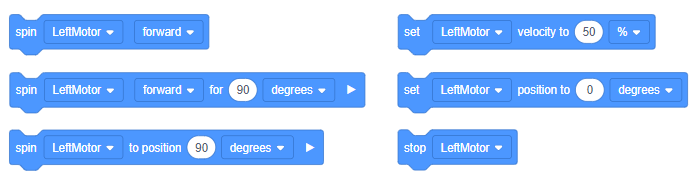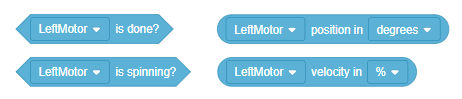# Coding with the IQ Smart Motor Encoders

Not only does the VEX IQ Smart Motor turn electrical energy into mechanical energy, as most motors do, it also has features that most motors do not have, which make it “smart.” One of its main features is the quadrature encoder.

Reports from the VEX IQ Smart Motor’s quadrature encoder make it possible to know:

• The direction of the motor’s turn (forward/reverse or open/closed)
• The position of the motor and the amount of the motor’s turn and position (either in turns or degrees)
• The speed of the motor’s turn (based on the encoder’s position data and tracked time)

Because the quadrature encoder reports the motor’s states, they can be used in behind-the-scenes calculations to make many of the Motion and Sensing blocks work within the VEXcode IQ Blocks programming language.

## Motion Blocks that use data from the quadrature encoderThe Motion blocks shown to the left use measures from the quadrature encoder as data to precisely control the behavior of the motor. The Left Motor is shown in all of the examples above but the same is true of any IQ Smart Motor.

Here are examples of how the quadrature encoder’s data are being used to make the Motion blocks work:

• The direction of turn: The encoder tracks whether the motor turns forward or in reverse so the programmer can set:
• The direction of the motor, like within the spin and spin for blocks

The measure of direction is also combined with the measure of speed when using the set velocity block because negative parameters set within the block have the motor spin in reverse. This is why it is called the set velocity block: it sets both the direction and speed and uses the encoder’s data to do so.

• The amount of turn: The encoder tracks how far the motor has turned and its current position so the programmer can set:
• The distance of the motor’s turn like within the spin for block
• A position to maintain when stopped as it does when using the stop block to ‘hold’ a position (requires first setting the stopping to ‘hold’)
• A position to spin to as it does when using the spin to position block. For this block, data about the amount of turn is used along with data about the direction of turns so that the spin to position block can determine the direction of rotation to reach the position set by the programmer
• The speed of turn: The encoder’s data can also be used to track the speed of the motor’s turns so the programmer can set:
• The speed like within the set motor velocity block

## Sensing Blocks that use data from the quadrature encoderThe Sensing blocks shown to the left highlight how data from the encoder are used to precisely report the behavior of the motor.

Here is how the quadrature encoder’s data are used for these four Sensing blocks:

• Is the motor spinning? (Motor is spinning block)
• If the encoder does not report a direction of turn or a change in position, then it must not be turning (0 = FALSE).
• If the encoder does report a directions of turn and a change in position, then it must be turning (1 = TRUE).
• Is the motor done? (Motor is done block)
• If the encoder does not report a direction of turn or a change in position, then it must be done (1 = TRUE).
• If the encoder reports a direction of turn and a change in position, then it must not be done yet (0 = FALSE).
• What is the motor’s velocity? (Velocity of motor block)
• The encoder’s data is used to track the speed of the motor’s turns and its direction. Reporting the velocity combines these two data points.
• The velocity is reported as either a +/- percentage (%) or a +/- revolutions per minute (rpm).
• What is the motor’s position? (Position of motor block)
• Data from the encoder can be used to report the motor’s position either in degrees or the number of turns.

In sum, the VEX IQ Smart Motor’s quadrature encoder tracks the motor’s states and reports data that can then be used to make the VEXcode IQ Blocks work easily for programmers.